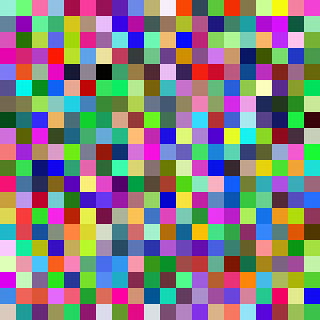This took me a while to figure out so I figured I better document it.

I’m working on a project that requires that I get the average color for a section of an image. This was the function I created to do that:

Unfortunately this wasn’t working and I was instead getting random colors returned. It certainly wasn’t the average color I was hoping for.

The problem lay in the last line (22). When you call the `color.RGBA()` method it takes a `uint8` and converts it to a `uint32`. The reasoning is that it avoids overflow if you are trying to do some kind of calculation on your RGB values. To do that conversion it first has to change the 8 bit value to a 16 bit value then from there it drops the 16 bit value into a 32 bit integer container. To convert from 8 to 16 bits it multiplies the RGB values by 0x101 meaning if you ever want to convert back down to 8 bit you need to divide by that same value.

So the return statement of my AverageImageColor function should look as follows:

Here’s the difference that makes in the image: